## what is 9/10 divided by 3.8 ? By quotient

Question

what is 9/10 divided by 3.8 ? By quotient

in progress 0
6 months 2021-08-18T07:44:46+00:00 1 Answers 19 views 0

## Answers ( )

1. Given: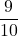divided by 3.8.

To find:

The quotient.

Solution:

We have,divided by 3.8.

Mathematically it can be written as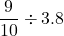Convert the decimal into fraction by multiplying and dividing by 10.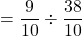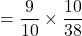[Reciprocal rule of division]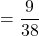(Cancel out the common factors)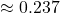Therefore, the quotient is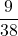, which is approximately 0.237.